#Function Repository Resource:

# ReflectPoints

Reflect a set of points using a line or plane defined by two or three points

Contributed by: Wolfram Staff
 ResourceFunction["ReflectPoints"][mirror,pts] reflects pts across mirror.

## Details and Options

ReflectPoints works on 2D and 3D.

## Examples

### Basic Examples

Reflect points about a given line:

 In:=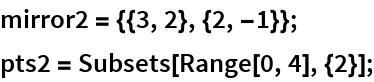In:=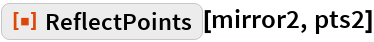Out=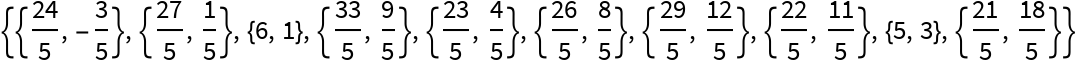In:=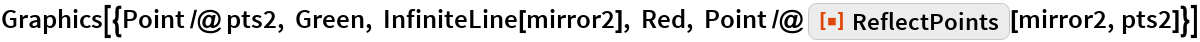Out=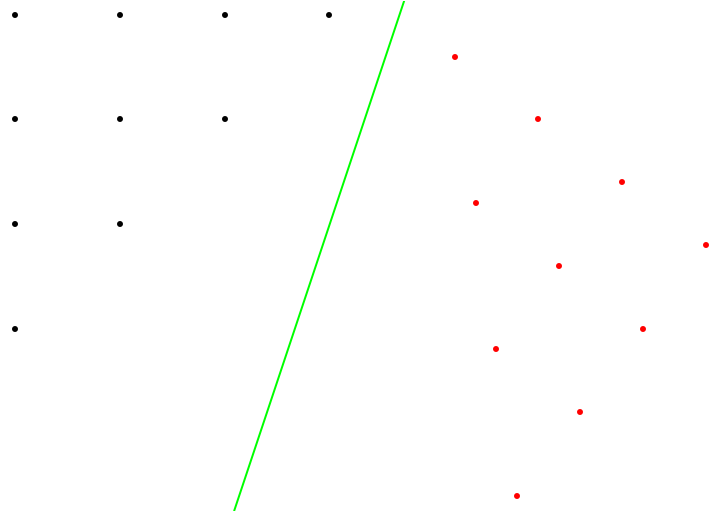Reflect points about a given plane:

 In:=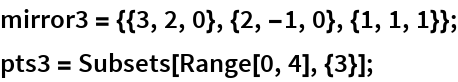In:=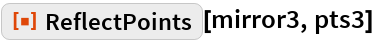Out=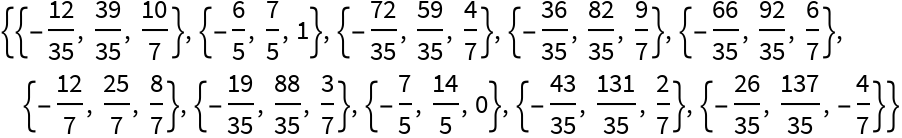In:=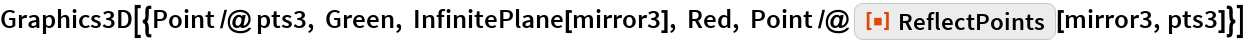Out=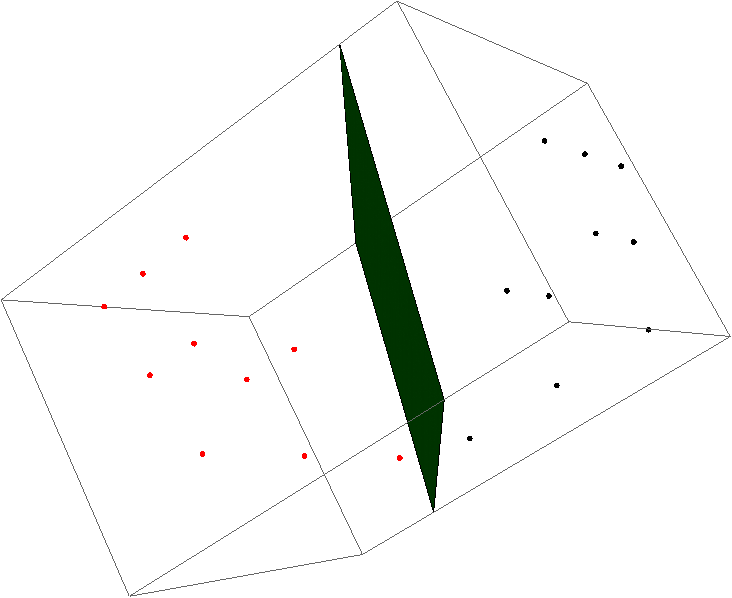## Requirements

Wolfram Language 11.3 (March 2018) or above